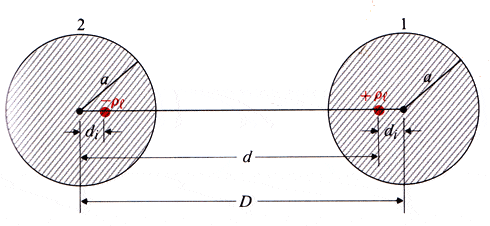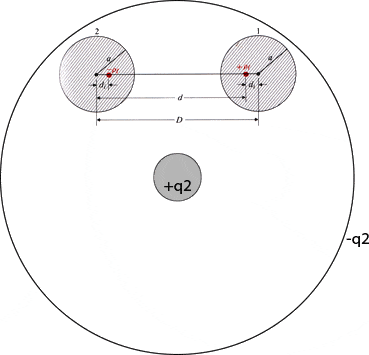# Image charges question

JerryG
I have the below figure I took from my text book from a section that explains how to calculate the capacitance of two transmission wires using image line charges.My question is that if I add two more conductors like shown below, how would it affect the line image charges? I'm not sure how to figure out where the image line charges would go, or if this can even be done analytically.We just studied image charges in lecture and I'm trying to understand what you are trying to do. Are those two conductors inside the insulation of a coaxial cable? If so is +q2 connected to 1 and -q2 connected to 2 somewhere at infinity (like the same terminal of a power source)?

JerryG
We just studied image charges in lecture and I'm trying to understand what you are trying to do. Are those two conductors inside the insulation of a coaxial cable? If so is +q2 connected to 1 and -q2 connected to 2 somewhere at infinity (like the same terminal of a power source)?

Conductors 1 and 2 are two conductors on the same voltage source. The other two, -q2 and +q2 on the coax are connected to a separate voltage source. So all four conductors have different potentials. I am trying to calculate the new capacitance of conductors 1 and 2 after adding the coax part, but I just don't know how to go about this. I'm not sure where I would put the line image charges to create the four equipotential surfaces.